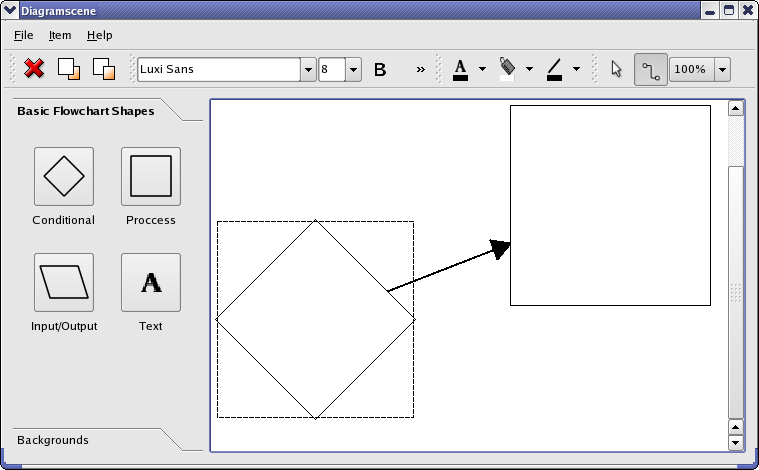# How to create an Arrow in QT

• I am trying to insert an customised QCPScatteredStyle in my QCustom plot for that i require an arrow , I have seen some samples but I was not able to understand them , Please any one provide me a simple sample to understand . And it would be better if it is developed in QPainterPath

• QPainterPath

Hi
What do you mean ?
How to draw an arrow using QPainter or something very tied to QCustomPlot ?

Arrow like this one ?• @mrjj Yes I want an arrow like this one

• You can reuse the arrow class/its Painter function that draws the arrow.

• @mrjj I saw the sample but i was not able to understand haw they have created the arrow thats why i asked for a simple sample

• @ManiRon
Ok.?
its just the paint function you need..

anyway, here it goes

``````void DrawLineWithArrow(QPainter& painter, QPoint start, QPoint end) {

painter.setRenderHint(QPainter::Antialiasing, true);

qreal arrowSize = 40; // size of head
painter.setPen(Qt::black);
painter.setBrush(Qt::black);

QLineF line(end, start);

double angle = std::atan2(-line.dy(), line.dx());
QPointF arrowP1 = line.p1() + QPointF(sin(angle + M_PI / 3) * arrowSize,
cos(angle + M_PI / 3) * arrowSize);
QPointF arrowP2 = line.p1() + QPointF(sin(angle + M_PI - M_PI / 3) * arrowSize,
cos(angle + M_PI - M_PI / 3) * arrowSize);

arrowHead << line.p1() << arrowP1 << arrowP2;
painter.drawLine(line);

}
... call like
DrawLineWithArrow(paint, QPoint(0, 0), QPoint(300, 300));
``````• DrawLineWithArrow(paint, QPoint(0, 0), QPoint(300, 300));

I tried to call like the way you mentioned (DrawLineWithArrow(paint, QPoint(0, 0), QPoint(300, 300));) but it is throwing error, Am i making any mistake ? This was the error

C:\Qt\Qt5.5.0\Examples\Qt-5.5\widgets\graphicsview\diagramscene\mainwindow.cpp:87: error: no matching function for call to 'MainWindow::DrawLineWithArrow(QPainter*&, QPoint, QPoint)'
DrawLineWithArrow(paint,QPoint(0,0), QPoint(0,0));
^

• @ManiRon

Hi
You could show how you defined "paint" as that could help.

If you call it from the painter function of the sample
where paint is a pointer you need
DrawLineWithArrow( * paint,QPoint(0,0), QPoint(330,330));

Or simply change it to take a pointer and not a ref (&)

If doubt plese show the complete code from where u call it.

• This post is deleted!

• @mrjj I Found the error i didnt define the paint correctly

• @mrjj

``````                #include "mainwindow.h"

#include "ui_mainwindow.h"

#include "qpainter.h"

#include "math.h"

#include "cmath"

#include "QPointF"

#include "QPolygonF"

MainWindow::MainWindow(QWidget *parent) :

QMainWindow(parent),

ui(new Ui::MainWindow)

{

ui->setupUi(this);

QPainter paint;

DrawLineWithArrow(paint,QPoint(0,0), QPoint(0,0));

}

MainWindow::~MainWindow()

{

delete ui;

}

void MainWindow::DrawLineWithArrow(QPainter& painter, QPoint start, QPoint end)

{

painter.setRenderHint(QPainter::Antialiasing, true);

qreal arrowSize = 40; // size of head

painter.setPen(Qt::black);

painter.setBrush(Qt::black);

QLineF line(end, start);

double angle = std::atan2(-line.dy(), line.dx());

QPointF arrowP1 = line.p1() + QPointF(sin(angle + M_PI / 3) * arrowSize,

cos(angle + M_PI / 3) * arrowSize);

QPointF arrowP2 = line.p1() + QPointF(sin(angle + M_PI - M_PI / 3) * arrowSize,

cos(angle + M_PI - M_PI / 3) * arrowSize);

arrowHead << line.p1() << arrowP1 << arrowP2;

painter.drawLine(line);

}
``````

This is my whole code , Now my doubt is where i will be able to see this arrow

• @ManiRon You need to overwrite paintEvent to be able to paint! https://doc.qt.io/qt-5/qwidget.html#paintEvent
"Now my doubt is where i will be able to see this arrow" - on the widget where you overwrite paintEvent.

• @jsulm I tried it on my Mainwindow and i was not able to see

• You need to overwrite paintEvent to be able to paint!

You need to overwrite paintEvent to be able to paint!

I am new to this thats why , can you please explain How this can be done ?

• @jsulm
Thanks Sir, I got it and made it, Here i provide my working code so that it will be useful for others

``````         #include "mainwindow.h"

#include "ui_mainwindow.h"

#include "qpainter.h"

#include "math.h"

#include "cmath"

#include "QPointF"

#include "QPolygonF"

#include "QPaintEvent"

MainWindow::MainWindow(QWidget *parent) :

QMainWindow(parent),

ui(new Ui::MainWindow)

{

ui->setupUi(this);

setBackgroundRole(QPalette::Base);

setAutoFillBackground(true);

}

MainWindow::~MainWindow()

{

delete ui;

}

void MainWindow::DrawLineWithArrow(QPainter& painter, QPoint start, QPoint end)

{

painter.setRenderHint(QPainter::Antialiasing, true);

qreal arrowSize = 40; // size of head

painter.setPen(Qt::black);

painter.setBrush(Qt::black);

QLineF line(end, start);

double angle = std::atan2(-line.dy(), line.dx());

QPointF arrowP1 = line.p1() + QPointF(sin(angle + M_PI / 3) * arrowSize,

cos(angle + M_PI / 3) * arrowSize);

QPointF arrowP2 = line.p1() + QPointF(sin(angle + M_PI - M_PI / 3) * arrowSize,

cos(angle + M_PI - M_PI / 3) * arrowSize);

arrowHead << line.p1() << arrowP1 << arrowP2;

painter.drawLine(line);

}

void MainWindow::paintEvent(QPaintEvent *)

{

static const QPoint points = {

QPoint(50, 100),

QPoint(20, 20),

};

QPainter painter(this);

painter.setPen(pen);

painter.setBrush(brush);

painter.setRenderHint(QPainter::Antialiasing, true);

qreal arrowSize = 40; // size of head

painter.setPen(Qt::black);

painter.setBrush(Qt::black);

QLineF line(points, points);

double angle = std::atan2(-line.dy(), line.dx());

QPointF arrowP1 = line.p1() + QPointF(sin(angle + M_PI / 3) * arrowSize,

cos(angle + M_PI / 3) * arrowSize);

QPointF arrowP2 = line.p1() + QPointF(sin(angle + M_PI - M_PI / 3) * arrowSize,

cos(angle + M_PI - M_PI / 3) * arrowSize);

arrowHead << line.p1() << arrowP1 << arrowP2;

painter.drawLine(line);

//    painter.setRenderHint(QPainter::Antialiasing, false);

//    painter.setPen(palette().dark().color());

//    painter.setBrush(Qt::NoBrush);

//    painter.drawRect(QRect(0, 0, width() - 1, height() - 1));

//    painter.restore();

}``````

• @jsulm One more doubt if i want to use QPainterpath to draw the same arrow how this can be done

Why I ask the same in QPainterPath is that i want to use it in QCustomplot

• QPainterPath

Yes i think you can convert the code to use QPainterPath .
it has a line function
https://doc.qt.io/qt-5/qpainterpath.html#lineTo
and a